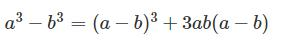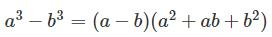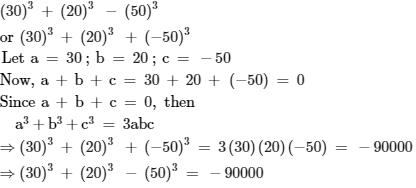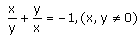# Test: Algebraic Identities Cubic Type

## 20 Questions MCQ Test Mathematics (Maths) Class 9 | Test: Algebraic Identities Cubic Type

Description
This mock test of Test: Algebraic Identities Cubic Type for Class 9 helps you for every Class 9 entrance exam. This contains 20 Multiple Choice Questions for Class 9 Test: Algebraic Identities Cubic Type (mcq) to study with solutions a complete question bank. The solved questions answers in this Test: Algebraic Identities Cubic Type quiz give you a good mix of easy questions and tough questions. Class 9 students definitely take this Test: Algebraic Identities Cubic Type exercise for a better result in the exam. You can find other Test: Algebraic Identities Cubic Type extra questions, long questions & short questions for Class 9 on EduRev as well by searching above.
QUESTION: 1

### Expansion and simplification of 8(3h - 4) + 5(h - 2) gives

Solution:

Now, 8 (3h - 4) + 5 (h - 2)

= 24h - 32 + 5h - 10, the expansion

= 24h + 5h - 32 - 10

= 29h - 42, the simplification

QUESTION: 2

### Evaluate (11)3​

Solution:

11³= (10+1)³=1000+1+30(11)=1001+330=1331

QUESTION: 3

### Factorise:(3x- 5y)3+ (5y – 2z)3 + (2z – 3x)3

Solution:

We don't have to cube them all to factorise. Now, One thing should be remembered that, in time of this type of factorisation we have to first add the polynomials to check if the sum is zero or not.If it is zero, then the factorisation will be in form of 3(abc). Now, lets sum them, (3x-5y) +(5y-2z) +(2z-3x).The answer is zero. So put the expression in form of 3(abc). So the factorisation of the expression is 3(3x-5y)(5y-2z)(2z-3x).

QUESTION: 4

Evaluate: 53 – 23 – 33

Solution:

53 - 23 - 33​​​​​​​
= 125 - 8 - 27
= 90

QUESTION: 5

Factorise : 8a3+ b3 + 12a2b + 6a b2

Solution:

8a3 + b3+ 12a2b + 6ab2
8a3 + b3+ 12a2b + 6ab2
= (2a)3 + (b)3 + 3(2a)(b) (2a + b)
= (2a + b)3 | Using Identity VI
= (2a + b)(2a + b)(2a + b)

QUESTION: 6

Evaluate (x+y+z)2

Solution:
QUESTION: 7

Factorise : 8a3 + b3 + 12a2 b + 6ab2

Solution:

8a3 + b3+ 12a2b + 6ab2
8a3 + b3+ 12a2b + 6ab2
= (2a)3 + (b)3 + 3(2a)(b) (2a + b)
= (2a + b)3 | Using Identity VI
= (2a + b)(2a + b)(2a + b)

QUESTION: 8

p3+ q3 + r3 – 3pqr = ?​

Solution:
QUESTION: 9

Factorize: 125a3 – 27b3 – 225a2b + 135ab2.​

Solution:
QUESTION: 10

Evaluate (104)3

Solution:

(104)³
=(100 + 4)³
Use ( a +b)³ = a³ + b³ + 3ab( a + b)
then,
( 100 + 4)³ = ( 100)³ +(4)³ +3(100)(4)(100+4)
=(10²)³ + 64 + 1200(104)
=1000000 + 64 + 124800
=1124864.

QUESTION: 11

Factorize: (x – y)3 + (y – z)3 + (z – x)3

Solution:
QUESTION: 12

Factorise: x7y + xy7

Solution:
QUESTION: 13

What is the value 83 – 33 (without solving the cubes)?​

Solution:

(8-3)((8)² + 8×3 + (3)²) = 5(64 + 24 + 9) = 5×97 = 485

QUESTION: 14

p3+ q3 = ?​

Solution:
QUESTION: 15

What is the value of 53 – 13(without solving cube)?

Solution:

53 - 13 can be solved using the identity ;

(a3 - b3) = (a - b) (a2 + b2 + ab)

53 - 13 = ( 5 - 1 ) ( 52 + 12 + 5*1)

=(4) (25 + 1 + 5)

= (4) (31)

= 124

QUESTION: 16

Factorize: 27x3 – 125y3

Solution:
QUESTION: 17

a3 – b3 equals​

Solution:

It is the identity equationIn the fraction equationQUESTION: 18

Evaluate: 303 + 203 – 503.​

Solution:

We have to find the value ofQUESTION: 19

If, the value of x3 – y3 is :

Solution:
QUESTION: 20

Factorization of 103²-9 yields

Solution: